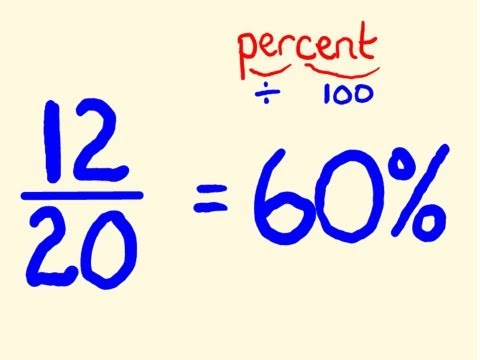# What is the EASIEST way to calculate percentages????

I’m so terrible at math and no matter how many times somebody explains it , i won’t ever understand how to calculate percentages. What is the EASIEST way to learn how?

Ex: What is 34% of 364

Things like that, everybody has their own way on explaining it and it never makes sense to me.

Relevance
• What does this have to do with personal finance?

• Try typing '34% of 364' into Google.

Voila.

• The way I do it is firstly look at the sum and guess roughly, 34% is about a third so looking at around 120, that gives me a guide, then I do 10 percent 3 times so 3 x 36.4 which is 109.2 then add 4 percent which is 14.56 giving you 123.76

• 1% of 100 is 1

10 % of 100 is 10

1% of 634 is 6.34

10% of 634 is 63.40

I hope this helps

• multiply. in your example == 34.0% ==> .34 (notice how the decimal point moved over 2 places) x 364. do on paper or a calculator.

Source(s): I like math. Go figure.
• Percent means divided by 100. "Percent of" means times 1/100. Example: 34% of 364 is 34 divided by 100 times 364 or 34/100 times 364.

• Anonymous
8 months agoDoesn't get any easier than this video IMO